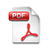On Auditing Elections When Precincts Have Different Sizes
Working Paper No.:  55
Date Published:  2008-11-30

#### Author(s):

Ronald L. Rivest, Massachusetts Institute of Technology

#### Abstract:

We address the problem of auditing an election when precincts may have different sizes, and suggest methods for picking a sample of precincts to audit that precinct size into account. One method yields optimal auditing strategies together with an exact measure of its effectiveness (probability of detecting corruption of a given size).

We restrict attention to basic auditing strategies, in which each precinct Pi is audited independently with some probability pi determined by the auditor. The auditing probability for a precinct will depend on the size of the precinct, with larger precincts audited more frequently; when all precincts have the same size they will have the same probability of being audited.

We first show how, given the auditor’s simpel auditing strategy, how to efficiently compute an optimal strategy for the adversary, using dynamic programming. This also yields the exact probability that the auditor will detect the adversary’s corruption in one or more precincts.

We then show how to embed the above procedure in an efficient iterative optimization computation that appears to always converge upon the optimal auditing strategy.

Finally, we present the “logistic auditing strategy,” which is very easy to compute and appears to yield optimal or nearly optimal auditing strategies when the amount of corruption being sought is noticeably larger than the maximum precinct size.

In the logistic approach, the auditor picks each precinct to be audited independently with a probability p that depends on the size v of the precinct (in votes), as follows: p = 1 − exp(−v/w) (1) where w is an adjustable globabl parameter that can be chosen to achieve a given confi- dence level (aka statistical power), to achieve a given expected number of precincts audited, or to achieve a given expected auditing workload. We call this approach a “logistic audit” since equation (1) is an instance of the familiar “logistic function” .

As a precinct gets larger, the probability that it is audited increases; as the precinct size passes the threshold w the probability of being audited passes 1 − 1/e ≈ 63%.

The logistic approach also enables estimation of the probability of detecting fraud: if the adversary corrupts precincts containing a total of at least C votes, then the auditor will detect fraud with probability at least 1 − exp(−C/w) . For example, by choosing w ≈ C/3, the auditor will detect fraud of magnitude C with probability at least 95%; choosing w ≈ C/4.605 gives a 99% confidence level (statistical power).

#### AttachmentOn Auditing Elections When Precincts Have Different Sizes  (Size: 206 KB)